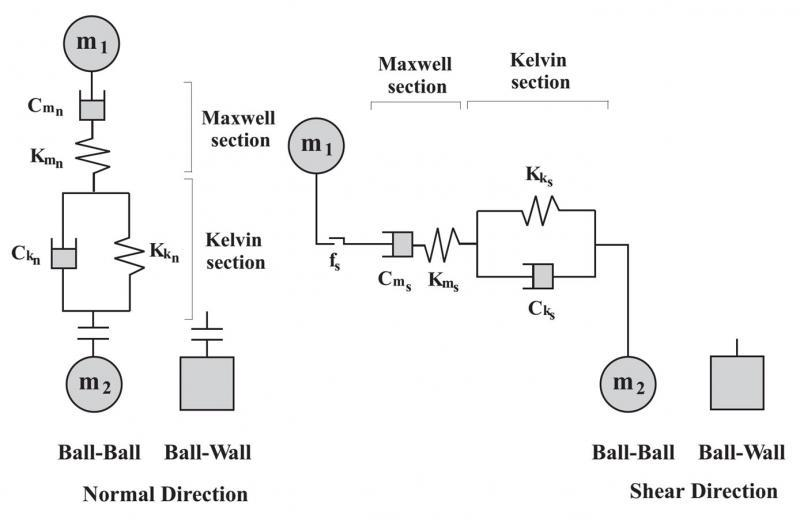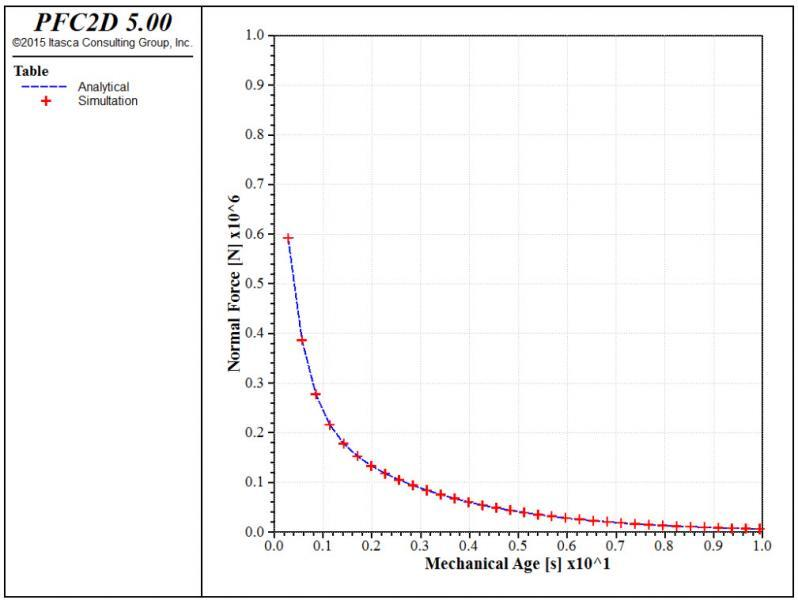## Burger's Model in PFC

### Introduction

The Burger's model provides a Kelvin model acting in series with a Maxwell model, in both the normal and shear direction. The Kelvin model is the combination of a linear spring and dashpot components that act in parallel with one another. The Maxwell model is the combination of a linear spring and dashpot components that act in series. The Burger's model act over a vanishingly small area, and thus transmit only a force.

### Behavior Summary

The rheological components of the Burger's model are shown in the figure below, for both the normal and shear directions. In both directions, the model combines a Kelvin and a Maxwell model acting in series. In the normal direction, the Kelvin model provides a linear spring with stiffness Kkn and a dashpot with viscosity Ckn, and the Maxwell model provides a linear spring with stiffness Kmn and a dashpot with viscosity Cmn. The Burger's model can sustain tensile forces (Mt =0) or not (Mt =1). In the shear direction, , the Kelvin model provides a linear spring with stiffness Kks and a dashpot with viscosity Cks, and the Maxwell model provides a linear spring with stiffness Kmsand a dashpot with viscosity Cms. A slider with friction coefficient fs limits the value of the shear force according to a Coulomb law.Rheological components of the Burger's model.

The following figure shows the time-decay of the normal force calculated by PFC2D, compared to the analytical solution for two balls fixed with a non-zero overlap. In both PFC2D and PFC3D models, the numerical solution shows that the contact force exponentially decreases as time increases, which coincides with the analytical solution shown by the blue dashed line in the figures.Time history of normal contact force of Burger's model. The simulation results are shown in red marks, the analytical solution with the blue dashed line (2D model).